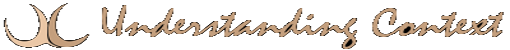# 01 Jan backpropagation

An algorithm that uses errors in the output (discrete differences between the correct output and the actual output) to train an artificial neural system to derive a correct output automatically.

Here’s an excerpt from Stanford Research Institute International:

## The Backpropagation Algorithm

1.
Propagates inputs forward in the usual way, i.e.

• All outputs are computed using sigmoid thresholding of the inner product of the corresponding weight and input vectors.
• All outputs at stage n are connected to all the inputs at stage n+1
2.
Propagates the errors backwards by apportioning them to each unit according to the amount of this error the unit is responsible for.

We now derive the stochastic Backpropagation algorithm for the general case. The derivation is simple, but unfortunately the book-keeping is a little messy.

•input vector for unit j (xji = ith input to the jth unit)
•weight vector for unit j (wji = weight on xji)
•, the weighted sum of inputs for unit j
• oj = output of unit j ()
• tj = target for unit j
• Downstream(j) = set of units whose immediate inputs include the output of j
• Outputs = set of output units in the final layer

Since we update after each training example, we can simplify the notation somewhat by imagining that the training set consists of exactly one example and so the error can simply be denoted by E.

We want to calculatefor each input weight wji for each output unit j. Note first that since zj is a function of wji regardless of where in the network unit j is located,Furthermore,is the same regardless of which input weight of unit j we are trying to update. So we denote this quantity by.

Consider the case when. We knowSince the outputs of all unitsare independent of wji, we can drop the summation and consider just the contribution to E by j.Thus(17)

Stanford Research Institute International

Now consider the case when j is a hidden unit. Like before, we make the following two important observations.

1. For each unit k downstream from jzk is a function of zj
2. The contribution to error by all unitsin the same layer as j is independent of wji

We want to calculatefor each input weight wji for each hidden unit j. Note that wji influences just zj which influences oj which influenceseach of which influence E. So we can writeAgain note that all the terms except xji in the above product are the same regardless of which input weight of unit j we are trying to update. Like before, we denote this common quantity by.

Also note thatand. Substituting,Thus,(18)

Stanford Research Institute International

We are now in a position to state the Backpropagation algorithm formally.

Formal statement of the algorithm:

Stochastic Backpropagation(training examples,ninhno)

Each training example is of the formwhereis the input vector andis the target vector.is the learning rate (e.g., .05). ninh and no are the number of input, hidden and output nodes respectively. Input from unit i to unit j is denoted xji and its weight is denoted by wji.

• Create a feed-forward network with ni inputs, nh hidden units, and no output units.
• Initialize all the weights to small random values (e.g., between -.05 and .05)
• Until termination condition is met, Do
• For each training example, Do
1. Input the instanceand compute the output ou of every unit.
2. For each output unit k, calculate3. For each hidden unit h, calculate4. Update each network weight wji as follows:This site uses Akismet to reduce spam. Learn how your comment data is processed.